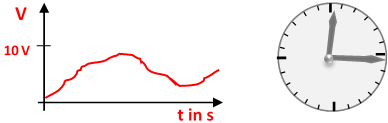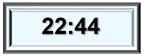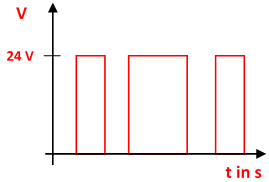### Analog and digitalExamples of analog quantities

In general, analog values ​​can take any value within a permissible range. Accordingly, an analog display must also give each value.Calculation frame

Countable elements represent digital values. Digital comes from 'digitus' (lat. Finger). A number can be represented by a number of fingers, for example. A simple digital calculator is the well-known calculation frame. Here a number is represented by the number of balls.

Digital values consist of countable elements!Digital clock

A numeric display is called a 'digital' display. Measuring instruments with a numerical display are called 'digital measuring instruments'; clocks with a numerical display are called digital clocks.

Note:Binary Signal

Computers and automation systems process electrical signals. One could represent the number 3, for example, by 3 electrical impulses. To represent the number 100,000 one would need 100,000 impulses. This makes no sense - numerical codes are used here.

#### Logical states of binary signals

Binary technology knows signals with two possible states. In other words: These elements are bivalent or binary. Examples of this are easy to find:

First binary state                  Second binary state
Switch closed                        Switch open
Pulse present                        Pulse not present
Voltage high                          Voltage low
Current does not flow         No current measurable

Since information is processed electronically, it must be represented by binary code. There are certain tolerances for these binary voltage states. The low voltage level is designated L (low); the higher voltage level is designated H (high). The terms "logic 0" and "logic 1" are also frequently used.